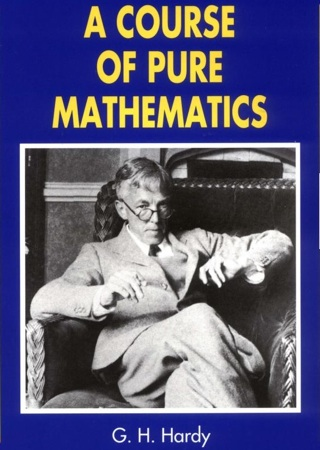# Arc length: the sad and intriguing case of the length of a circle

Posted by: Gary Ernest Davis on: February 16, 2011

When I was a little fellow in secondary school I was puzzled by my teacher imagining string placed around a circle and straightening the string to find it’s length. I was also very uneasy about Ï€ as the ratio of the circumference ofÂ  circle to its diameter.

For some time all I had was a vague feeling of unease aboutÂ Ï€ and lengths of circles.Then, at about age 16, I read Godfey Hardy’s “A Course of Pure Mathematics” in which, as I recall, he stated that in order to find the length of a circle we first had to show that a circle HAD a length.

This, of course, presupposes an advanced mathematical concept: the length of a curved line, in the plane, or in space.

The reason this is an “advanced” concept is not because it is especially difficult, but because it is based on a mathematical definition, and not taken for granted as something that is perceptually obvious.

When I understood this, I knew why my teacher’s string straightening worried me: how did he know that the straightening operation preserved length, if he could not even say what the length of a curved line was?

Of course, my teacher took the string straightening exercise as his informal definition of the length of a curved line. But it seemed to me, even as a young fellow, that the basis of mathematical reasoning could not, or should not, lie in manipulating pieces of string.

Well, the definition of the length of a curved line stems from taking a (finite) number of points on the curved line and joining them, in order, by straight line segments:

These straight line segments taken together, give us a broken line approximation to the curve:

Now we can calculate the length of the broken line by adding together the lengths of the individual straight line segments.

What is the length of the curve that the broken lineÂ  approximates?

The idea is to take more and more points on the curved line, and see if the lengths of the corresponding broken lines approach some definite number.

If they do that number is called the length of the curved line.

In other words, we find the length of a curved line by approximating it by broken lines and seeing how the lengths of these broken lines behave.

In more advanced mathematics this involves a process of integration, in which the major difficulty is the idea of a limit.

It is VERY important that we choose points actually on and not just near, the curved line to join together. Even for a straight line segment, let alone a curved one, things can go badly wrong if we choose points close to, but not actually on the line whose length we are trying to find.

As an example, think about approximating the hypotenuse of the following right triangle by broken lines:

If we use points not exactly on the red line, but close, we get a length of the broken line that is not even close to the length of the red line:

If we repeat this process, halving the black line segments each time, we get a total length of the broken line equal to 2, at each step, but the blue dots get as close as we like to the red line.

It is very important, therefore, in obtaining broken line approximations to a curve, to find the length of the curve, that we choose endpoints of the segments actually ON the curve and not merely close.

#### Length of a circle

A circle is a curve, so how can we can we use this concept of length to find the length of a circle, say a circle of radius 1?

Of course we approximate a circle by polygons, with corner points placed ON the circle:

This is what Archimedes did to approximate the area of a circle.

But at each step of our approximation we are only ever approximating the length of the circle, not actually finding it.

So, at no stage do we ever actually know what is the length of a circle of radius 1.

Following Euler we now call this length 2Ï€. But that’s just a name.

Do we actually “know” what is the value of Ï€?

To paraphrase a well known and recent US President, it depends what we mean by “know”.

If we mean, can we exactly locate Ï€ as a decimal number, the answer is: “No”.